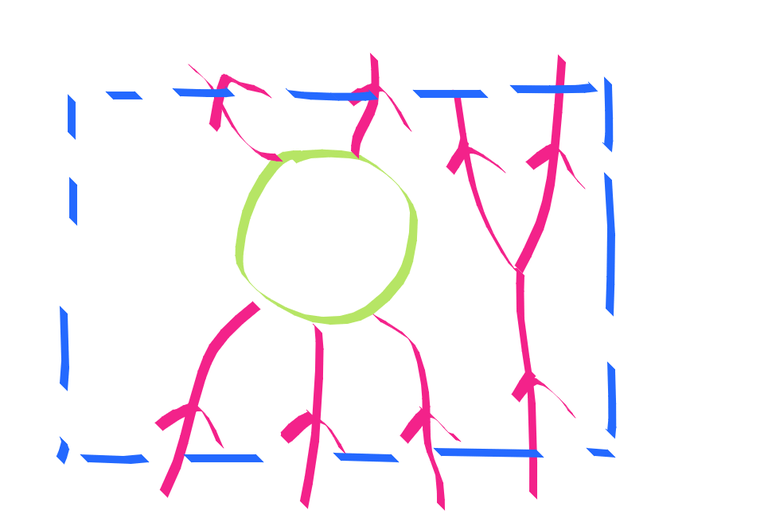Petri nets are a framework for modeling dynamical systems that is very intuitive to some people. The vanilla version is that there are discrete tokens at nodes on a graph representing resources of some kind and tokens can be combined according to the firing of transition rules into new tokens in some other location.

This is a natural generalization of chemical reaction kinetics, where tokens are particular kinds of atoms that need to come together. It also is a useful notion for computer systems, where tokens represent some computational resource.

To me, this becomes rather similar to a flow problem or circuit problem. Tokens feel a bit like charge transitions are a bit like current (although not necessarily conservative). In a circuit, one can have such a small current that the particulate nature of electric current in terms of electrons is important. This happens for shot noise or for coulomb blockade for example.

If the number of tokens is very large, it seems intuitively sensible to me that one can well approximate the behavior by relaxing to a continuum. Circuits have discrete electrons and yet are very well approximated by ohm’s laws and the like. Populations are made of individuals, and yet in the thousands their dynamics are well described by differential equations.

It seems to me that mixed integer programming is a natural fit for this problem. Mixed integer programming has had its implementations and theory heavily refined for over 70 years so now very general purpose and performant off the shelf solvers are available. The way mixed integer programs are solved is by considering their quickly solved continuous relaxation (allowing fractional tokens and fractional transitions more akin to continuous electrical circuit flow) and using this information to systematically inform a discrete search process. This seems to me a reasonable starting approximation. Another name for petri nets is Vector Addition Systems, which has more of the matrix-y flavor.

We can encode a bounded model checking for reachability of a petri net into a mixed integer program fairly easily. We use 2-index variables, the first of which will be used for time step. We again turn to the general purpose functional way of encoding pointful dsls into pointfree ones as I have done here and here. The key point is that you need to be careful where you generate fresh variables. This is basically copy catting my posts here. http://www.philipzucker.com/categorical-combinators-for-graphviz-in-python/ http://www.philipzucker.com/a-sketch-of-categorical-relation-algebra-combinators-in-z3py/

I’m like 70% sure what I did here makes sense, but I’m pretty sure the general idea makes sense with some fiddling.

T = 10 # total number of time steps as a global parameter
class PetriCat():
def compose(f,g):
def res():
a, b , fcon = f()
b1, c, gcon = g()
return a, c, fcon + gcon + [b == b1]
def idd():
def res()
x = cvx.Variable((T-1,1), integer = True)
return x, x, [x >= 0]
def par(f,g):
def res():
a, b , fcon = f()
c, d , gcon = g()
return cvx.vstack([a,c]), cvx.vstack([b,d]), fcon + gcon
return res
def weighted_block(wi, wo, wint):
def res():
(Na, Ni) = wi.shape # number inputs,  actions
(Na1,No) = wo.shape
(Na2, Nint) = wint.shape
assert(Na == Na1)
assert(Na == Na2)
action = cvx.Variable((T-1, Na), integer=True)
internal = cvx.Variable((T, Nint), integer =True)
flowin = action @ wi
flowout = action @ wo
return flowin, flowout, [internal[1:,:] == internal[:-1,:] + action @ wint, action >= 0, internal >= 0]
return res
def run(f):
a, b, fcon = f()
prob = cvx.Problem(cvx.Minimize(1), fcon)
prob.solve()
return a, b

# We need some way of specifying the initial and final states of things, Just more parameters to constructor functions I think


The big piece is the weighted_block function. It let’s you build a combinator with an internal state and input and output flow variables. You give matrices with entries for every possible transition. Whether transitions occurred between $t$ and $t+1$ is indicated by integer variables. There is also possible accumulation of tokens at nodes, which also requires integer variables. Perhaps we’d want to expose the token state of the nodes to the outside too?Weighted block schematically looks something like this

We can also get out a graphical representation of the net by reinterpreting our program into GraphCat. This is part of the power of the categorical interface. http://www.philipzucker.com/categorical-combinators-for-graphviz-in-python/

When I talked to Zach about this, he seemed skeptical that MIP solvers wouldn’t eat die a horrible death if you threw a moderately large petri net into them. Hard to say without trying.

#### Thoughts

There is an interesting analogy to be found with quantum field theory in that if you lift up to considering distributions of tokens, it looks like an occupation number representation. See Baez. http://math.ucr.edu/home/baez/stoch_stable.pdf

If you relax the integer constraint and the positivity constraints, this really is a finite difference formulation for capacitor circuits. The internal states would then be the charge of the capacitor. Would the positivity constraint be useful for diodes?

I wonder how relevant the chunky nature of petri nets might be for considering superconducting circuits, which have quantization of quantities from quantum mechanical effects.

Cvxpy let’s you describe convex regions. We can use this to implement a the convex subcategory of Rel which you can ask reachability questions. Relational division won’t work probably. I wonder if there is something fun there with respect the the integerizing operation and galois connections.

Edit: I should have googled a bit first. https://www.sciencedirect.com/science/article/pii/S0377221705009240 mathemtical programming tecchniques for petri net reachability. So it has been tried, and it sounds like the results weren’t insanely bad.